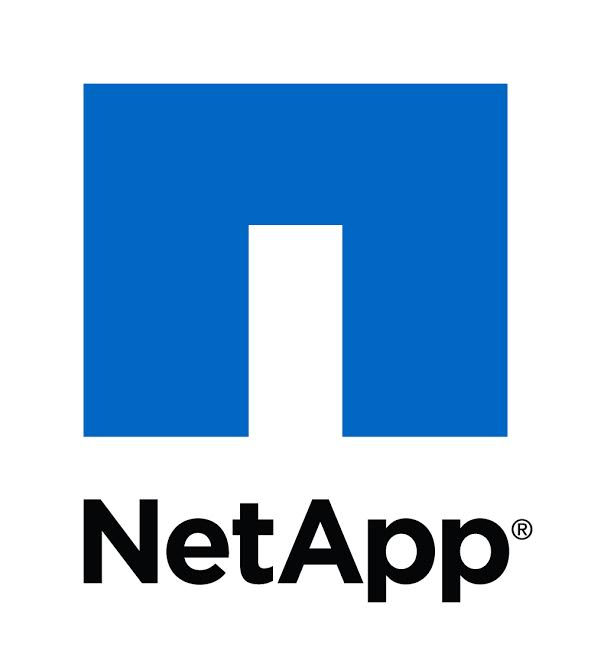Flight Tickets
Tag(s):

Algorithms, Depth First Search, Graph, Hard

Problem
Editorial
Analytics

There are $N$ cities in the country Byteland. Each city has some special value associated with it. All the cities are connected to one another either via direct flights or a series of connecting flights. There are a total of $N-1$ flights available and it is always possible to reach any city from any other city thus the structure of flights between the cities in Byteland forms a tree. The fare of flight between two cities is given by the distance between the two cities in the tree.

Now Rohan books flights between the two cities if and only if their special value is equal. He wants to book the cheapest and distinct $K$ flights. He follows the following rules while booking flights-

• Two flights are distinct if their start city or destination city is different from other booked flights.
• Note that since Byteland is mysterious, booking of flight from the same city to the same city is also possible with a cost of 0.
• If Rohan books a flight from the city $A$ to city $B$ then he can't book any flight from the city $B$ to city $A$.
• In the output, you need to print the price of the last flight that he would book. If it is impossible to book $K$ flights then you need to print $-1$.

Input Format :

The first line contains two space-separated integers $N$ and $K$ denoting total number of cities and the total flights that Rohan needs to book. Each of the next $N-1$ lines contain $2$ space separated integers $u$ and $v$ whcih denote that there exists a flight between the cities with number $u$ and $v$. The next line contains $N$ space-separated integers, where the $i^{th}$ integer denotes $C[i]$ which denotes the special value associated with the city numbered $i$.

It is guaranteed the given input edges form a valid tree.

Output Format :

Print a single integer denoting the answer.

Constraints :

$1 \le N \le 3 \times 10^4$

$1 \le C[i] \le 10^9$

$1\le K \le (n \times (n-1))/2$

SAMPLE INPUT
5 6
1 2
2 3
3 4
4 5
1 1 1 1 1

SAMPLE OUTPUT
1

Explanation

There are 5 flights with cost 0 i.e. each from the city to itself. Now the sixth flight is of cost 1.

Time Limit: 3.0 sec(s) for each input file.
Memory Limit: 256 MB
Source Limit: 1024 KB
Marking Scheme: Marks are awarded when all the testcases pass.
Allowed Languages: Bash, C, C++, C++14, Clojure, C#, D, Erlang, F#, Go, Groovy, Haskell, Java, Java 8, JavaScript(Rhino), JavaScript(Node.js), Julia, Kotlin, Lisp, Lisp (SBCL), Lua, Objective-C, OCaml, Octave, Pascal, Perl, PHP, Python, Python 3, R(RScript), Racket, Ruby, Rust, Scala, Swift, Swift-4.1, TypeScript, Visual Basic

CODE EDITOR

Initializing Code Editor...

Contributor